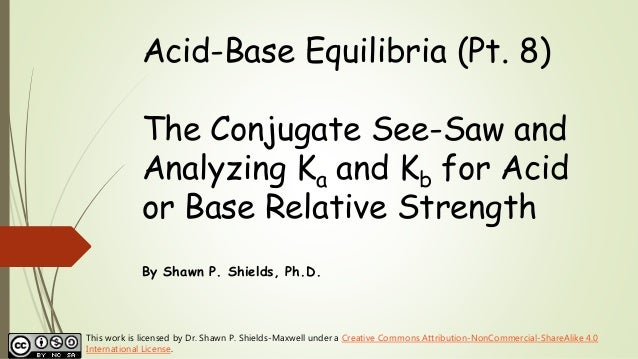Successfully reported this slideshow.
We use your LinkedIn profile and activity data to personalize ads and to show you more relevant ads. You can change your ad preferences anytime.Upcoming SlideShare
Loading in …5
×
• Full Name
Comment goes here.

Are you sure you want to Yes No
Your message goes here• Be the first to comment

### Chem 2 - Acid-Base Equilibria VIII: The Conjugate See-Saw and Analyzing Ka and Kb for Acid or Base Relative Strength

1. 1. Acid-Base Equilibria (Pt. 8) The Conjugate See-Saw and Analyzing Ka and Kb for Acid or Base Relative Strength By Shawn P. Shields, Ph.D. This work is licensed by Dr. Shawn P. Shields-Maxwell under a Creative Commons Attribution-NonCommercial-ShareAlike 4.0 International License.
2. 2. Recall: Conjugate Acid-Base Pairs Acetic acid (CH3COOH) and its conjugate base… acetic acid conjugate base for acetic acid (acetate) “loseable” H+
3. 3. Recall: Ka and Kb for Conjugate Acid-Base Pairs 𝐊 𝐚 ∙ 𝐊 𝐛 = 𝐊 𝐖 acetic acid acetate (base) Ka = 1.76  10-5 Kb = 5.68  10-10
4. 4. Analyzing the Magnitude of Ka The relative strength of the weak acid (compared to others weak acids) can be determined by comparing Ka values. If Ka is relatively large, the weak acid is relatively strong.
5. 5. Analyzing the Magnitude of Ka A larger Ka means the weak acid has a larger percent dissociation (more products). 𝐇𝐀 𝐚𝐪 + 𝐇 𝟐 𝐎 𝐥 ⇌ 𝐇 𝟑 𝐎+ 𝐚𝐪 + 𝐀− (𝐚𝐪) 𝐊 𝐚 = 𝐇 𝟑 𝐎+ 𝐀− 𝐇𝐀 𝐩𝐫𝐨𝐝𝐮𝐜𝐭𝐬 𝐫𝐞𝐚𝐜𝐭𝐚𝐧𝐭𝐬
6. 6. Example: Analyzing Ka Values Which is the stronger weak acid? HF Ka = 3.5  10-4 CH3COOH Ka = 1.76  10-5 Ka ∝ 𝐩𝐫𝐨𝐝𝐮𝐜𝐭𝐬 𝐫𝐞𝐚𝐜𝐭𝐚𝐧𝐭𝐬
7. 7. Example: Analyzing Ka Values Which is the stronger weak acid? HF Ka = 3.5  10-4 CH3COOH Ka = 1.76  10-5 A larger Ka value means the acid is more dissociated (stronger). Ka ∝ 𝐩𝐫𝐨𝐝𝐮𝐜𝐭𝐬 𝐫𝐞𝐚𝐜𝐭𝐚𝐧𝐭𝐬
8. 8. Example: Comparing pKa Values How does pKa relate to weak acid strength? HF Ka = 3.5  10-4 CH3COOH Ka = 1.76  10-5 Calculate pKa =  log Ka
9. 9. Example: Comparing pKa Values HF Ka = 3.5  10-4 pKa =  log (3.5  10-4) = 3.46 CH3COOH Ka = 1.76  10-5 pKa =  log (1.76  10-5) = 4.75 Lower pKa means stronger weak acid!
10. 10. Central Concept: The Conjugate See-Saw The stronger the acid, the weaker the conjugate base. The stronger the base, the weaker the conjugate acid.
11. 11. Conjugate See-Saw Example: Comparing Ka and Kb Values For HF, the acid dissociation constant Ka is 3.5  10-4. What is the conjugate base? Calculate Kb for the conjugate base, and explain what that value tells you.
12. 12. Conjugate See-Saw Example: Comparing Ka and Kb Values HF Ka = 3.5  10-4. Kb for the conjugate base F… 𝐊 𝐛 = 𝐊 𝐖 𝐊 𝐚 = 𝟏. 𝟎 × 𝟏𝟎−𝟏𝟒 𝟑. 𝟓 × 𝟏𝟎−𝟒 = 𝟐. 𝟗 × 𝟏𝟎−𝟏𝟏 Ka is much larger than Kb, so F is a much weaker base than HF is an acid (i.e., it’s a very wimpy base.)
13. 13. Conjugate See-Saw Example 2: Comparing Ka and Kb Values For NH4 +, the acid dissociation constant Ka is 5.6  10-10. What is the conjugate base? Calculate Kb for the conjugate base, and explain what that value tells you.
14. 14. Conjugate See-Saw Example 2: Comparing Ka and Kb Values NH4 + Ka = 5.6  10-10. Kb for the conjugate base NH3 (ammonia) 𝐊 𝐛 = 𝐊 𝐖 𝐊 𝐚 = 𝟏. 𝟎 × 𝟏𝟎−𝟏𝟒 𝟓. 𝟔 × 𝟏𝟎−𝟏𝟎 = 𝟏. 𝟖 × 𝟏𝟎−𝟓 Kb is much larger than Ka NH4 + is a much weaker acid than NH3 is a base (i.e., it’s a very wimpy acid.)
15. 15. Conjugate See-Saw for Strong Acids Strong acids dissociate completely in water. 𝐇𝐂𝐥 𝐚𝐪 + 𝐇 𝟐 𝐎 𝐥 → 𝐇 𝟑 𝐎+ 𝐚𝐪 + 𝐂𝐥− (𝐚𝐪) The conjugate base of a strong acid does not affect the pH of the water. The conjugate base is NOT basic in water.
16. 16. Conjugate See-Saw for Strong Bases Strong bases dissociate completely in water. 𝐍𝐚𝐎𝐇 → 𝐍𝐚+ 𝐚𝐪 + 𝐎𝐇− (𝐚𝐪) The conjugate acid of a strong base does not affect the pH of the water. The conjugate acid is NOT acidic in water.
17. 17. Conjugate See-Saw Summary Acid/Conjugate Base If the Ka for the weak acid is relatively large, the Kb for the conjugate base will be relatively small. If the weak acid is relatively strong, the conjugate base will be very weak.
18. 18. Conjugate See-Saw Summary Base/Conjugate Acid If the Kb for the weak base is relatively large, the Ka for the conjugate acid will be relatively small. If the weak base is relatively strong, the conjugate acid will be very weak.
19. 19. Next up, Salts that Hydrolyze in Water to Affect pH (Pt 9)